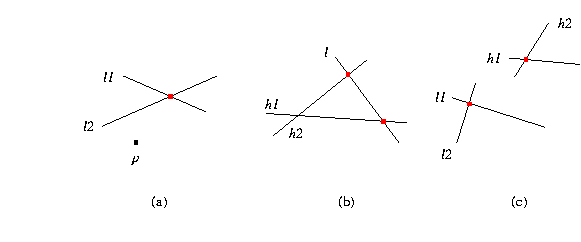## CGAL::compare_y

Depending on which CGAL kernel is used, different versions of this global function are available. This is described below.

With the basic 2D and 3D Kernel (see Chapter 7)

 Comparison_result compare_y ( Point_2 p, Point_2 q) compares Cartesian y-coordinates of p and q. Comparison_result compare_y ( Point_3 p, Point_3 q) compares Cartesian y-coordinates of p and q.Comparison_result compare_y ( Point_2 p, Line_2 l1, Line_2 l2) compares the y-coordinates of p and the intersection of lines l1 and l2, see (a) in the figure above. Comparison_result compare_y ( Line_2 l, Line_2 h1, Line_2 h2) compares the y-coordinates of the intersection of line l with line h1 and with line h2, see (b) in the figure above. Comparison_result compare_y ( Line_2 l1, Line_2 l2, Line_2 h1, Line_2 h2) compares the y-coordinates of the intersection of lines l1 and l2 and the intersection of lines h1 and h2 , see (c) in the figure above.

With the 2D Circular Kernel (see Chapter 9)

#include <CGAL/global_functions_circular_kernel_2.h>

If this kernel is used, in addition to the function and the combination of 2D types described above, another version of the function is provided.

 Comparison_result compare_y ( Circular_arc_point_2 p, Circular_arc_point_2 q) compares the y-coordinates of p and q. Comparison_result compare_y ( Circular_arc_point_2 p, Point_2 q) compares the y-coordinates of p and q.

With the 3D Spherical Kernel (see Chapter 10)

#include <CGAL/global_functions_spherical_kernel_3.h>

If this kernel is used, in addition to the function and the combination of 2D types described above, another version of the function is provided.

 Comparison_result compare_y ( Circular_arc_point_3 p, Circular_arc_point_3 q) compares the y-coordinates of p and q. Comparison_result compare_y ( Circular_arc_point_3 p, Point_3 q) compares the y-coordinates of p and q.